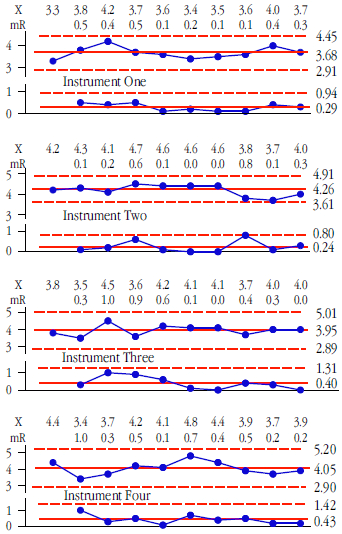# Content By James Beagle IIIBy: James Beagle III, Donald J. Wheeler

In Parts One and Two we defined the equivalence of instruments in terms of bias and measurement error based on studies using a single standard. Here we look at comparing instruments for differences in bias or differences in measurement error while using multiple standards.By: Donald J. Wheeler, James Beagle III

Last month we provided an operational definition of when measurement systems are equivalent in terms of bias. Here we will look at comparing the within-instrument measurement error between two or more systems.

Once again we must emphasize that it makes no sense to seek to compare measurement systems that do not display a reasonable degree of consistency. Consistency must be demonstrated, it cannot be assumed, and a consistency chart is the simplest way to do this.Figure 1: Consistency charts for instruments 1, 2, 3, & 4By: Donald J. Wheeler, James Beagle III

As soon as we have two or more instruments for measuring the same property the question of equivalence raises its head. This paper provides an operational definition of when two or more instruments are equivalent in practice.

Churchill Eisenhart, Ph.D., while working at the U.S. Bureau of Standards in 1963, wrote: “Until a measurement process has been ‘debugged’ to the extent that it has attained a state of statistical control it cannot be regarded, in any logical sense, as measuring anything at all.” Before we begin to talk about the equivalence of measurement systems we need to know whether we have yardsticks or rubber rulers. And the easiest way to answer this question is to use a consistency chart.By: Donald J. Wheeler, James Beagle III

Whenever we make a measurement, we have to decide how many digits to record. Traditional answers for this question are often little more than guesswork glorified by time. And with digital readouts, are all the displayed digits real? This column provides a sound and practical answer to these perennial questions.

There are two uncertainties that affect every measurement. These uncertainties are the round-off and measurement error. The round-off has to do with the uncertainty introduced by the choice of measurement increment. Measurement error is the inherent uncertainty introduced by the measurement system itself. To understand the impact of each of these uncertainties we begin by looking at them separately.

### Round-off uncertainty

Assume that the only uncertainty in our measurements is the round-off. Since we only record observations to a finite number of decimal places, there will only be a finite number of possible values for our measurements. These possible values will always end up being some integer multiple of the measurement increment (MI). If we denote our observed value as X, then X will be some multiple of MI.By: Donald J. Wheeler, James Beagle III

Sometimes we use a chart for individual values and a moving range (an XmR chart) to assess the homogeneity of a finite data set. Since this is an "off-label" use for the XmR chart, we first consider the drawbacks associated with using a sequential technique as a one-time test, and then present an adaptation of the X chart (the analysis of individual values or ANOX) that functions like other one-time statistical tests.

### The XmR chart

Like all process behavior charts, the XmR chart was designed for the sequential analysis of a continuing stream of observational data. Here the data will generally represent one condition, and the purpose of the chart is to identify unplanned changes in the underlying process. After the baseline period, where we compute the limits, we extend the limits forward and continue to add data to the chart. Each time we add an additional point to the chart we are performing an act of analysis. Each of these analyses asks if the current value is consistent with the baseline period. And, as with all sequential procedures, we want to perform each of these acts of analyses in a conservative manner in order to reduce the overall risk of a false alarm.